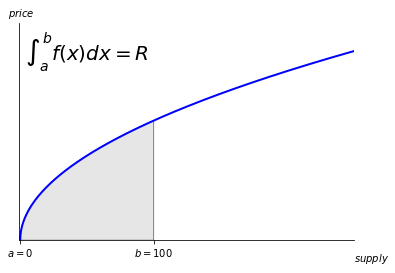# Bonding Curves

At the heart of Strata is the ability to buy and sell a new token without a liquidity provider. This is accomplished via a Bonding Curve. A Bonding Curve is an Automated Market Maker (AMM) that sets the price of a token relative to the supply of that token. The result is similar to how Poker Chips work at a Casino -- put USD into the cash register and you get some chips. Give the chips back, and you get USD back. The USD in the cash register always matches the supply of chips. The main difference is that the chips get more expensive as the number circulating increases.

Every bonding curve consists of a Base Token (USD in our poker example) and a Target token (the chips). The Base token is the token we set the price relative to, the Target token is the token the Automated Market Maker sells.

A simple example might be

$P = 0.01 S$

This means that the price when there is one token in circulation is

$0.01 * 1 = 0.01$

The price when there are 100 is

$0.01 * 100 = 1$

When a Target token is purchased, the price in Base tokens goes into a Reserve account (the cash register). When a Target token is sold, it is burned and Base tokens are returned from the Reserve

## Pricing the Bonding Curve​

You can find the price for some number of tokens $n$ at any given supply $S_0$ by taking the area under the curve (don't worry, the sdk takes care of this for you)

$P(n) = \int_{S_0}^{S_0 + n} 0.01 S dS$

Visualizing this calculation, we can see the reserves, $R$, of the Base token when the supply of the target token is $100$ on the curve $P = \sqrt{S}$This pricing assumes that the reserves do not change outside of buying and selling. But what happens if someone were to burn tokens without asking for any reserves, or directly send tokens to the reserve? Find out more in Advanced Bonding Curves

## Royalties​

The owner of a bonding curve may extract royalties on both buying and selling, both in terms of Base and Target tokens.

For example, a bonding curve owner bonding SOL to COIN may take a 5% royalty of SOL for every purchase of COIN. They could also take a 5% royalty in terms of COIN for every purchase.

The destination of these royalties are configurable.

# Token Bonding SDK

The Token Bonding SDK has five main commands: initializeCurve, createTokenBonding, updateTokenBonding, buy, sell.

## Curves​

To create a bonding curve, first we must specify the formula for this curve. This is done via initializeCurve

Let's start with a simple exponential curve. Exponential curves are modeled as

$P = c (S^{\frac{pow}{frac}}) + b$

Let's create a simple curve

$0.01 \sqrt{S}$
import { ExponentialCurveConfig } from "@strata-foundation/spl-token-bonding";

We can fetch that curve:

What if we want a curve that changes over time? Perhaps it starts out at a constant price, then after 2 days changes into an exponential function.

$t_0 = S$
$t_1 = S^2$
import { ExponentialCurveConfig, TimeCurveConfig } from "@strata-foundation/spl-token-bonding";

## Bonding​

Using our curve from above, let's create a simple bonding curve against SOL:

import { getAssociatedAccountBalance } from "@strata-foundation/spl-utils";import { PublicKey } from "@solana/web3.js";

Now fetch that bonding:

Now, we can buy and sell on that curve:

Now fetch the balance:

Now fetch the balance again:

## Pricing​

We can get the prices behind a bonding curve by using getPricing

We can pass royalty accounts to createTokenBonding. Since we already have a bonding curve from above, let's use updateTokenBonding instead.